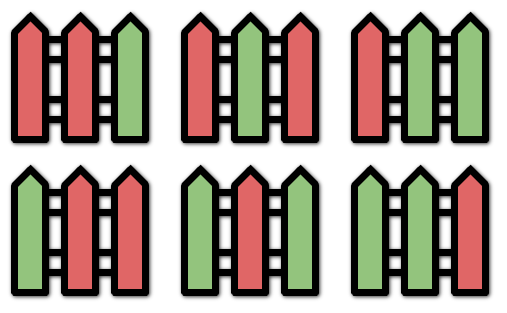### LeetCode• ㊗️
• 大家
• offer
• 多多！

## Problem

You are painting a fence of n posts with k different colors. You must paint the posts following these rules:

Every post must be painted exactly one color. There cannot be three or more consecutive posts with the same color. Given the two integers n and k, return the number of ways you can paint the fence.

Example 1:Input: n = 3, k = 2
Output: 6


Explanation: All the possibilities are shown. Note that painting all the posts red or all the posts green is invalid because there cannot be three posts in a row with the same color.

Example 2:

Input: n = 1, k = 1
Output: 1


Example 3:

Input: n = 7, k = 2
Output: 42


## Code

class Solution {
public int numWays(int n, int k) {
if(n <= 0 || k <= 0) return 0;

if(n == 1) return k;
if(n == 2) return k * k;

int[] dp = new int[n];

dp = k;
dp = k * k;

for(int i = 2; i < n; i++){
dp[i] = dp[i - 1] * (k - 1) + dp[i - 2] * (k - 1);
}

return dp[n - 1];
}
}

class Solution:
def numWays(self, n: int, k: int) -> int:
if n == 0:
return 0
if n == 1:
return k
if n == 2:
return k * k
dp =  * n
dp = k
dp = k * k

for i in range(2, n):
# 涂上和之前第一个post不同的颜色
# 涂上和之前第二个post不同的颜色
dp[i] = dp[i - 1] * (k - 1) + dp[i - 2] * (k - 1)

return dp[n - 1]